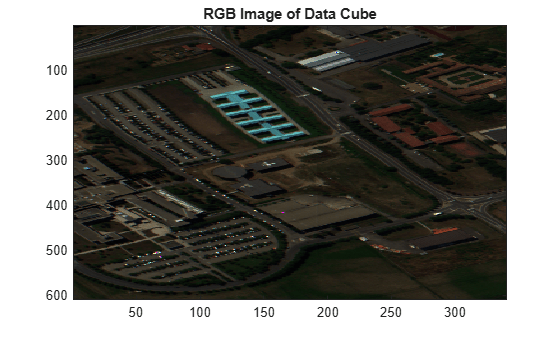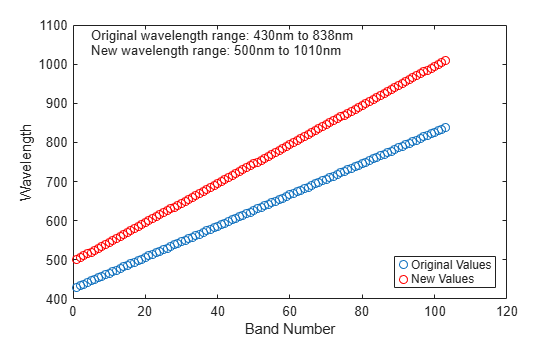# hypercube

## Description

The `hypercube` function reads hyperspectral data and returns an `hypercube` object. The object contains the hyperspectral data cube and its related properties. Use the object functions to remove or select a desired hyperspectral band, assign new pixels values, generate colored image, and write hyperspectral data to the ENVI (environment for visualizing images) file format.

## Creation

### Syntax

``hcube = hypercube(filename)``
``hcube = hypercube(img,hdr)``
``hcube = hypercube(___,wavelength)``
``hcube = hypercube(tifFile,wavelength)``
``hcube = hypercube(image,wavelength)``
``hcube = hypercube(image,wavelength,metadata)``

### Description

example

````hcube = hypercube(filename)` reads hyperspectral data from the specified input file `filename`. The input file can be a national imagery transmission format (NITF) file, Hyperion level 1R (L1R) file stored in hierarchical data format (HDF), ENVI header or image file, or metadata text extension (MTL) file that contains satellite data from earth observing (EO) satellites. EO-1 HyperionEO-1 Advanced Land Imager (EO-1 ALI)Landsat Multispectral Scanner (Landsat MSS)Landsat Thematic Mapper (Landsat TM)Landsat Enhanced Thematic Mapper Plus (Landsat ETM+) Landsat Operational Land Imager / Thermal Infrared Scanner (Landsat OLI / TIRS) NoteThe `hypercube` function reads satellite data that are stored in georeferenced tagged image file format (GeoTIFF). ```

example

````hcube = hypercube(img,hdr)` reads hyperspectral data from the data file `img`. The data file can be an ENVI image file or Hyperion L1R file. The function uses the metadata in the header file `hdr` to interpret the data from `img`. ```

example

````hcube = hypercube(___,wavelength)` specifies the wavelength for each spectral band in the input data and sets the `Wavelength` property of the output `hypercube` object.```
````hcube = hypercube(tifFile,wavelength)` reads hyperspectral data from a tagged image file format (TIFF) file `tifFile`.```

example

````hcube = hypercube(image,wavelength)` creates a `hypercube` object from the hyperspectral data cube `image` and the specified center wavelength values `wavelength`.```

example

````hcube = hypercube(image,wavelength,metadata)` creates a `hypercube` object from the hyperspectral data cube `image`, specified center wavelength values `wavelength`, and the metadata `metadata`. You can use this syntax to modify the `Metadata` property of a hypercube object. NoteThis function requires the Image Processing Toolbox™ Hyperspectral Imaging Library. You can install the Image Processing Toolbox Hyperspectral Imaging Library from Add-On Explorer. For more information about installing add-ons, see Get and Manage Add-Ons. ```

### Input Arguments

expand all

Input file name, specified as a character vector or string scalar. The input file name must be one of these file types.

 File Format Extensions Additional Requirements NITF files `.ntf``.nsf` None GeoTIFF metadata files from EO satellites `.txt` File name must end with suffix "MTL". ENVI image filesENVI header files `.dat`, `.img`, and `.raw``.hdr` Image and header file must be in the same folder and have the same file name. Hyperion level 1R image filesHyperion header files ```.L1R ````.hdr` Image and header file must be in the same folder and have the same file name.

Data Types: `char` | `string`

Image file name, specified as a character vector or string scalar. The input file must be a flat binary raster file with the `.dat`, `.raw`, or `.L1R` file extensions. The binary data must be in band sequential (BSQ), band-interleaved-by-pixel (BIP), or band-interleaved-by-line (BIL) format.

Data Types: `char` | `string`

Header file name, specified as a character vector or string scalar. The header file contains the metadata for the image file `img` and has the extension `.hdr`.

Data Types: `char` | `string`

Center wavelength values of each spectral band, specified as C-element vector. C is the spectral dimension, defined as the number of spectral bands, of the input hyperspectral data.

Data Types: `single` | `double` | `int8` | `int16` | `int32` | `int64` | `uint8` | `uint16` | `uint32` | `uint64`

TIFF file name, specified as a character vector or string scalar. The file name must include the extension `.tiff` or `.tif`.

Data Types: `char` | `string`

Input hyperspectral data, specified as a 3-D numeric array of size M-by-N-by-C. M and N are the number of rows and columns in the hyperspectral data, respectively. C is the number of spectral bands in the hyperspectral data.

Data Types: `single` | `double` | `int8` | `int16` | `int32` | `int64` | `uint8` | `uint16` | `uint32` | `uint64`

Metadata of hyperspectral data, specified as a structure array.

Data Types: `struct`

## Properties

expand all

Hyperspectral data cube, stored as a 3-D numeric array of size M-by-N-by-C. The data cube stores the hyperspectral data read from a file or numeric array as an array of 2-D monochromatic images. C is the number of images or spectral bands, M and N are the spatial resolution of the images in pixels. The data cube is of the same size and data type as the input data.

Data Types: `single` | `double` | `int8` | `int16` | `int32` | `int64` | `uint8` | `uint16` | `uint32` | `uint64`

Center wavelength values of each spectral band, specified as a C-element vector. C is the spectral dimension, defined as the number of spectral bands, of the input hyperspectral data. You can set this property by using `wavelength` input argument.

Data Types: `single` | `double` | `int8` | `int16` | `int32` | `int64` | `uint8` | `uint16` | `uint32` | `uint64`

Metadata of hyperspectral data, stored as a structure array with these fields as defaults.

FieldDescription
`Height`Height of the image or number of rows in the data cube, specified as a positive integer
`Width`Width of the image or number of columns in the data cube, specified as a positive integer
`Bands`Number of spectral bands comprising the data cube, specified as a positive integer
`DataType`

Data type of data, specified as any of these values:

• `"single"`

• `"double"`

• `"uint8"`

• `"uint16"`

• `"int16"`

• `"uint32"`

• `"int32"`

• `"uint64"`

• `"int64"`

`Interleave`

Data interleave, specified as any one of these values:

• `"bsq"` — Band-sequential

• `"bil"` — Band-interleaved-by-line

• `"bip"` — Band-interleaved-by-pixel

`HeaderOffset`

Zero-based location of the first element in the image file, specified as a positive integer

The header offset represents the number of bytes from the beginning of the image file to the start of the image data. The default value is 0.

`ByteOrder`Endianness of the data, specified as the string `"ieee-le"` for little endian or `"ieee-be"` for big endian.
`WavelengthUnits`Units for the wavelengths of spectral bands, specified as a string. The default value is `"Nanometers"`.

Note

The `Metadata` property of `hypercube` object can have one or more additional fields depending on the parameter values stored in the header file of the input hyperspectral data. You can modify the parameters values of the `Metadata` property or add new `Metadata` to the `hypercube` object by specifying the input argument `metadata`.

Data Types: `struct`

## Object Functions

 `assignData` Assign new data to hyperspectral data cube `cropData` Crop regions-of-interest `enviwrite` Write hyperspectral data to ENVI file format `selectBands` Select most informative bands `removeBands` Remove spectral bands from data cube `colorize` Estimate color image of hyperspectral data

## Examples

collapse all

Read hyperspectral data stored in the ENVI format into the workspace. Create a `hypercube` object by specifying an ENVI data file and the associated ENVI header file.

`hcube = hypercube('paviaU.dat','paviaU.hdr');`

Display the properties of the `hypercube` object.

`hcube`
```hcube = hypercube with properties: DataCube: [610×340×103 double] Wavelength: [103×1 double] Metadata: [1×1 struct] ```

Estimate an RGB image from the hyperspectral data by using the `colorize` function. Visualize the RGB image.

```rgbImg = colorize(hcube,'Method','RGB'); figure imagesc(rgbImg) title('RGB Image of Data Cube')```Inspect the metadata of the `hypercube` object.

`hcube.Metadata`
```ans = struct with fields: Filename: "Y:\jobarchive\Bspkg20b\2020_06_16_h06m34s27_job1406120_pass\matlab\toolbox\images\supportpackages\hyperspectral\hyperdata\paviaU.hdr" FileModDate: "25-Feb-2020 14:29:34" FileSize: 654 Format: "HDR" FormatVersion: '' SensorType: [0×0 string] Description: [0×0 string] AcquisitionTime: [0×0 string] RasterFormat: "ENVI" Height: 610 Width: 340 Bands: 103 DataType: "double" Interleave: "bsq" HeaderOffset: 0 ByteOrder: "ieee-le" BandNames: [0×0 string] FWHM: [] Gain: [] Offset: [] ReflectanceGain: [] ReflectanceOffset: [] BadBands: [] CloudCover: [] SunAzimuth: [] SunElevation: [] ```

Read ENVI format data into the workspace by specifying a header file that contains information about hyperspectral data. The associated ENVI binary data file must be stored in the same folder as the ENVI header file.

`hcube = hypercube('paviaU.hdr');`

Display the properties of the `hypercube` object.

`hcube`
```hcube = hypercube with properties: DataCube: [610×340×103 double] Wavelength: [103×1 double] Metadata: [1×1 struct] ```

Estimate an RGB image from the data cube by using the `colorize` function. Increase the contrast of the RGB image using contrast stretching. Visualize the RGB image.

```rgbImg = colorize(hcube,'Method','RGB','ContrastStretching',true); figure imagesc(rgbImg) title('RGB Image of Data Cube')```Assign new center wavelength values for the hyperspectral data. The number of wavelength values must be equal to the number of bands in the hyperspectral data cube. Each wavelength value must be unique values.

```minWavelength = 500; maxWavelength = 1010; newWavelength = minWavelength:5:maxWavelength;```

Create a new `hypercube` object with the new wavelength values.

`newhcube = hypercube('paviaU.hdr',newWavelength);`

Plot the old and the new wavelength values. Display the wavelength range.

```figure plot(hcube.Wavelength,'o') hold on plot(newhcube.Wavelength,'or') xlabel('Band Number') ylabel('Wavelength') str1 = ['Original wavelength range: ' num2str(min(hcube.Wavelength)) 'nm to ' num2str(max(hcube.Wavelength)) 'nm']; text(5,1075,str1) str2 = ['New wavelength range: ' num2str(min(newhcube.Wavelength)) 'nm to ' num2str(max(newhcube.Wavelength)) 'nm']; text(5,1035,str2) legend('Original Values','New Values','Location','SouthEast')```Read an RGB image into the workspace. An RGB image contains three spectral channels: red, green, and blue channels.

`image = imread('peppers.png');`

Specify the center wavelength values for the red, green, and blue channels as 700, 530, and 470 nanometers (nm) respectively.

`wavelength = [700 530 470];`

Create a `hypercube` object using the image and the wavelength values.

`hcube = hypercube(image,wavelength)`
```hcube = hypercube with properties: DataCube: [384×512×3 uint8] Wavelength: [3×1 double] Metadata: [1×1 struct] ```

Read a hyperspectral data into the workspace and inspect its properties.

`hcube = hypercube('paviaU.dat');`

Inspect the `Metadata` property of the `hypercube` object.

`hcube.Metadata`
```ans = struct with fields: Filename: "/mathworks/devel/bat/Bdoc22a/build/matlab/toolbox/images/supportpackages/hyperspectral/hyperdata/paviaU.hdr" FileModDate: "25-Feb-2020 03:59:34" FileSize: 654 Format: "HDR" FormatVersion: '' SensorType: [0x0 string] Description: [0x0 string] AcquisitionTime: [0x0 string] RasterFormat: "ENVI" Height: 610 Width: 340 Bands: 103 DataType: "double" Interleave: "bsq" HeaderOffset: 0 ByteOrder: "ieee-le" BandNames: [0x0 string] FWHM: [] Gain: [] Offset: [] ReflectanceGain: [] ReflectanceOffset: [] BadBands: [] CloudCover: [] SunAzimuth: [] SunElevation: [] SolarIrradiance: [] EarthSunDistance: [] WavelengthUnits: "Nanometers" ```

Find and remove the empty fields from the metadata.

```metadata = hcube.Metadata; fields = fieldnames(metadata); indx = find(structfun(@isempty,metadata)==1); newMetadata = rmfield(metadata,fields(indx));```

Set the value for the `AcquistionTime` field to current date.

```currentDate = string(datetime("now",Format="yyyy-MM-dd")); newMetadata.AcquistionTime = currentDate;```

Create a `hypercube` object with the new metadata. The `DataCube` and `Wavelength` properties of the new `hypercube` object is same as that of the input data.

`nhcube = hypercube(hcube.DataCube,hcube.Wavelength,newMetadata);`

Inspect the `Metadata` property of the new `hypercube` object.

`nhcube.Metadata`
```ans = struct with fields: Height: 610 Width: 340 Bands: 103 DataType: "double" Interleave: "bsq" HeaderOffset: 0 ByteOrder: "ieee-le" AcquistionTime: "2022-02-26" WavelengthUnits: "Nanometers" ```

## Version History

Introduced in R2020a